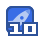### 论文研究-时滞Hopfield神经网络的随机稳定性分析.pdf收藏

T-S模型提供了一种通过模糊集和模糊推理将复杂的非线性系统表示为线性子模型的方法。研究了时滞Hopfield神经网络的随机稳定性（SFVDHNNs）。首先描述了SFVDHNNs模型，然后用Lyapunov方法研究了SFVDHNNs全局均方指数稳定性，通过可以被一些标准的数值分析方法求解的线性矩阵不等式（LMIs）得出了稳定性标准。
622008,44(16) Computer Engineering and Applications计算机工程与应用 n,下面条件成立,则(8)是全局方差稳定的: 非递减的,并且对所有的u∈R,g(u)≤1∧βlul,连接权矩阵要 Y PB 0 I=BP -nex+C3 求满足b=∑l,1≤i≤n,甚至满足对称性。这些限制性很强 O+C 的条件限制了在这些文献中提出的标准的应用。然而在本文 其中,Y=PA-AP+Q1+KQ2+C,=1-。 中,去掉了激励函数和连接权矩阵上的限制条件,只假设激励 证明由∏<0,可以保证存在一个足够小的数,使得 函数g是 Lipschitz连续的(H1)。另外本文还考虑到了连接权 PB 0 矩阵的特征,连接权矩阵可以表示出兴奋和抑制效果之间的差 ∑=BP-mQ2+C3 0≤0 别。因此,本文的结果比原来的更宽松。 举例说明:令r=2,看以下 SFVDHNNS的规则: 0 其中,三=BP-PA-AP+e"g1+ekQ2K+C1实际上,很明显地,对 规则i:如果()是m1,…,O(t)是mn,则dx(t)=[-42x(t) 所有的B≥0,△=BP+(c-1)Q1+(e9-1)kQK≥0。另外,有三=+Bfx(t-r(t)+0(x(t),x(t=7(1),t)do(t),=1,2,…,其中 r+△,因此就存在一个B>0使得∑≤0。 7是一个模糊集,0(1)=(61(t),…,0(1)是前提变量向量,n(t) 定义一个 Lyapunov- Krasovsky函数v(x(1),1)∈C2(Rx是标量布朗运动, R+;R+) fx)=x+1|-r-1 V(x(t),t=eBx(tPx(t)+ B(s+)x(s)O(s t-lt σ1(x,y,t)=(0.5x(t-r(t),0.4x2(t-r(t))r B(stfT(x(s))02f(x(s))d (10) σ2(x,y,t)=(0.2x1(t-r(t),0.3x2(t-7(t)) 有了这个公式,经过系统(8)就可以得到v(x(t),t)的导数: 很显然K=diag(1,1)和r=1,=(1/2)分别满足假设H1和H2, 这样对所有的ij=1,2,都有trce(o(x,y,t)o1(x,y,1)≤ dv(x(1),t)≤∑h(B(t)e×Bx(t)P2(1)+ex()Qx(t) 0.25x1(-r(t)+0.16x2(t-r())。 (1-5)x(t-T(t)OKx(t-T(t))+ePx(t)KO2Kx(t SFVDHNNS就可以被描述如下: (1-2)f(x(t-T(t)))22f(x(t-T(t)))+x'(t)(-PAFAP)x(t)+ (t)PB f(x(t-T(t)))+f(x(t-T(t)))B Px(t)+ dx(v=>h(6(D))IG-A x(t)+B: (x(t-r(t)))di+o (x(o), x(t x(t)Cu(t)+x(t-T())Cxx(t-T(t))+ T(t)), t)dw(t) f(x(t-7(1)))C3f(x(1-r(1)d+M(t) 60 50 令A= B A B 则存在 04 06 e"∑(6(1)1(x(=r(1)x∑(x(-r(1)d+M()(1) C1=C3=0,C2=diag(0.25,0.16),P=diag(1,1)>0,Q1= (t-r(t)) x(t-7(t)) 其中,M(1)=e∑h(6(t)()Po(x(t),x(t-r(t),t)+ o (x(t),x(t-T(L)), t)Px(t)]dw(t) 说定理1的条件是满足的,因此上述 SFVDHNNS系统是全局 既然∑≤0,就有EV(x(t),t)≤EV(0),从式(10)可以得到 02人0,使得定义在定理1上的矩阵是负定的也就是 差指数稳定的 EV(O((P)+-[(21+A(KOK)JElldpll 4结语 另一方面,从V(x(t),t)的定义,又可以得到 本文针对 SFVDHNN系统模型,首先通过使随机时滞 EV(x(t), t)>(P)e Ell ll2 (13) Hopfield神经网络作为T-S模型推理规则的后件,扩展了一般 综合式(12)(13),很容易得到:El(t,d)甲≤αeEb|2,其中的T-S模型;然后又通过 Lyapunov Krasovski方法研究了 A(P)+-[(21)+A(KQ2K)I SFVDHNN的全局方差指数稳定性,并提出一种判断标准,该 ,所以, SFVDHNNS(8)就是标准的稳定性条件是根据LM的解表示出来的。本文结果显 示,人们可以很广泛地选用激励函数,包括 Sigmoid函数和分 全局方差指数稳定的,定理得证 段线性函数。 说明2在本文中,通过构建一个合适的 Lyapunov Krasovskii函数,给出了一个充分条件来保证 SFVDHNN系统参考文献: [1 Hopfield J J Neural networks and physical systems with emergent 容易被检验的LMIs来表示出来的,因此可以被有效地用在 collect computational abilities J Proc Nat Acad Sci USA, 1982, 79 SFVDHNNS系统的设计和应用方面。 说明3当r=1时,系统(8)退化为随机时滞 Hopfield神经21 Neurons with graded response have collective computational prop- 网络,同样地,稳定性的充分条件也可以用在这种神经网络上。 erties like those of two-state neurons[ Proc Nat Acad Sci USA 在文献[1,2,11,14也针对带常量时延的随机 Hopfield神经网 1984,81:3088-3092 络提出了一些稳定性条件。在这些论文中,激励函数g假设为 下转82页)

...展开详情

2019-09-08
weixin_38744207•至尊王者

成功上传501个资源即可获取论文研究-时滞Hopfield神经网络的随机稳定性分析.pdf 12积分/C币 立即下载
1/312积分/C币 立即下载 ＞